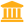Accueil  >>  Physics of continuous media
• Unité d'enseignement : Physics of continuous media
Nombre de crédits de l'UE : 6
Code APOGEE : PHY1011M
Responsabilité de l'UE :
CAUPIN FREDERIC
frederic.caupinuniv-lyon1.fr
04.72.44.85.65
Type d'enseignement
Nb heures *
Cours Magistraux (CM)
30 h
Travaux Dirigés (TD)
30 h
Travaux Pratiques (TP)
0 h
Durée de projet en autonomie (PRJ)
0 h
Durée du stage
0 h
Effectif Cours magistraux (CM)
210 étudiants
Effectif Travaux dirigés (TD)
35 étudiants
Effectif Travaux pratiques (TP)
18 étudiants

* Ces horaires sont donnés à titre indicatif.

Compétences attestées (transversales, spécifiques) :
Non rédigé
Programme de l'UE / Thématiques abordées :
Version anglaise de physique des milieux continus

Based on a scale separation (both in space and time) and on a local thermodynamic equilibrium hypothesis (to define local intensive observables like temperature, pressure or internal energy), Physics of Continuous Media aims at establishing the laws of behavior of physical quantities considering averages (density, concentration fields, …). Resulting continuous models are based on exact conservation laws and phenomenological relations derived from the linear response theory.

This lecture is made of three parts using the formalism described above : (i) diffusion processes, transport mechanisms, (ii) physical hydrodynamics to describe fluids under stress which flows as opposed to solids, (iii) elastic behavior of solids.

1 Introduction

2 Diffusion processes

Conservation equations for diffusion, thermal diffusion (Fourier laws), particle diffusion (Fick law), relaxation of a point source distribution,Brownian motion, Langevin equation, fluctuation-dissipation theorem, Einstein formula, diffusion in an external field

3 Physical hydrodynamics

Kinematic properties, convective derivative, stress rate tensor, conservation laws (mass, momentum, energy), Stress tensor, Newtonian regime and Navier-Stokes equation, Boundary conditions, Perfect fluids (Bernouilli equation), Flows (d’Alembert paradox), low Reynolds number flows, (Stokes problem), lubrication, wetting hydrodynamics

4  Linear elasticity

This part uses the formalism introduced and developed for hydrodynamics in the third part.

Elementary deformations, linear elasticity general theory (stress and deformation tensors, Hooke law), Isotropic media, equilibrium equation (Navier equation), Applications of the linear elasticity theory in the homogeneous and isotropic case (point force, Herz contact)

Modalités de contrôle des connaissances et Compétences 2022/23 :
TypeLibelléNatureCoef.
CTCT : Continuous mediaEpreuve écrite (tablette, QCM)-
Date de la dernière mise-à-jour : 18/04/2018